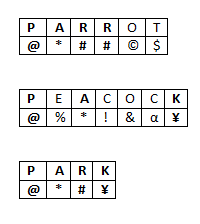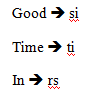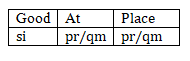# RRB ALP 2018 Practice Test Papers | Reasoning Questions (Day-46)

Dear Aspirants, Here we have given the Important RRB ALP & Technicians Exam 2018 Practice Test Papers. Candidates those who are preparing for RRB ALP 2018 can practice these Reasoning Questions to get more confidence to Crack RRB 2018 Examination.

[WpProQuiz 2583]

Click “Start Quiz” to attend these Questions and view Solutions

1. Rakshan travels 15 kms towards east, then turns right and travels 7 kms. He then turns right and travels 15 kms. How far is Rakshan from the starting point?
1. 15 km
2. 10km
3. 7km
4. 9km
1. In a certain code, if SQUARE is written as UQSERA, the TRIANGLE will be written as
1. AIRTELGN
2. ELGNAIRT
3. ARITELGN
4. ATRILGNE

3.Select the Missing number from the given responses.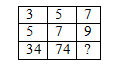1. 130
2. 140
3. 120
4. 110
1. If + denotes , denotes × , × denotes , and ÷ denotes +. Then find the value of 7 ÷ 11 – 4 + 2 × 10
1. 10
2. 19
3. 15
4. 20

5.Find the missing series

11, 24, 50, ____, 141, 206

1. 90
2. 80
3. 89
4. 91
1. C is grandmother of H. F is daughter of D. E is sister of F. G is Son of E. How H is related to G?
1. Brother
2. Sister
3. Cousin
4. Brother in law
1. Statement

Some mobiles are smart phones

Some smart phones are iphone

No iphone is Laptop.

Conclusion

No Laptop is Smart Phone

Some Iphones are Smartphone

1. Only I follows
2. Only II follows
3. Either I or II follow
4. Neither I nor II follows⨯
1. If Parrot is coded as @*##©\$, Peacock is coded as @%*!&α¥, then PARK is Coded as
1. @*#¥
2. *¥#\$
3. ¥*@\$
4. @#¥*
1. Question (9 – 10)

In a certain code language

“si rs ti” stands for “Good in Time”

“th si rs” stands for “every Good Time” and

“si pr qm” stands for “Good at place”

What would” si” stands for?

1. Time
2. Good
3. Place
4. Every
1. What would “Place” stands for?
1. pr
2. qm
3. Either (a) or (b)
4. Neither (a) or (b)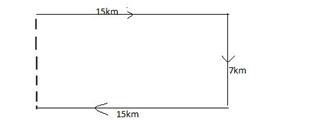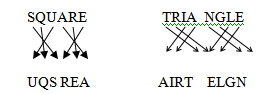32+ 52 = 9+25 = 34

52 + 72= 25+ 49= 74

72+ 92= 49+ 81 = 130

7 ÷ 11 – 4 + 2 × 10

As coded

7 + 11 × (4 ÷2) – 10 = 7 +  11×2 −10

= 7 + 22 – 10 =29 −10=19

11, 24, 50, X, 141, 206

11+13=24

24+26=50

50+39= 89

89+52=141

141+65=206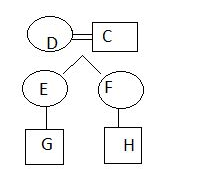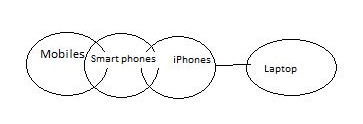No Laptop is Smart Phone.

Some Iphones are Smartphone is follows.